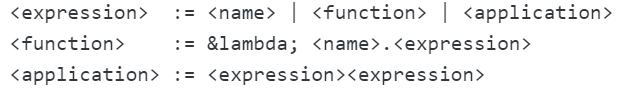# Lambda Calculus: Brief Overview

Lambda calculus (λ calculus) is a simple and practical system made up of two rules: a transformation rule and a function definition scheme. Any computable function can be expressed using these basic rules. It is used extensively in higher-order logic and computer programming, where it forms the underpinnings of many computer programs (like LISP).

It was developed in 1930 by Alonzo Church (Alan Turing’s doctoral advisor) to formalize the idea of effective computability. The λ symbol arose because the original notation (a caret “^” above the first parameter) was difficult to type, so it was replaced with the Greek letter Λ, which looks similar to a caret.

## The Expression

The expression is the core of λ calculus. The basic form is:

lambda [list of parameters].[body of function]

The body of function is the rules for calculating the value of the function.

The expression can be defined recursively as (Rojas, 1998):Where name (or variable) is a letter a, b, c,….

## Format

Lambda calculus can handle any calculable function, including very basic ones. For example, the square of a number is written as: λ x . x*x.

A second example, using a familiar algebraic formula:And let’s say you wanted to solve it for a= 2 and b = 5. In λ calculus, you would write that as:
ab.)2 5

This might seem like a more complicated way to write expressions, but it isn’t. That’s because, while I used 2 and 5 as a simple example to show you how the expression works, λ calculus doesn’t actually deal with numbers. It deals with functions, which don’t have to be named if you use λ-calculus for programming. Another benefit to λ is that while many programming languages require function values to be elements of a set, the value of a function in λ calculus can also be a function itself.

## References

Barendregt. H. (2012). The Lambda Calculus. Its Syntax and Semantics (Studies in Logic) Paperback. College Publications.
Lynn, B. Lambda Calculus. Retrieved April 14, 2020 from: https://crypto.stanford.edu/~blynn/lambda/
Rojas, R. (1997). A Tutorial Introduction to the Lambda Calculus. Retrieved April 14, 2020 from: https://personal.utdallas.edu/~gupta/courses/apl/lambda.pdf
The Lambda Calculus, Retrieved April 14, 2020 from: https://www.sjsu.edu/faculty/watkins/lambda.htm

CITE THIS AS:
Stephanie Glen. "Lambda Calculus: Brief Overview" From StatisticsHowTo.com: Elementary Statistics for the rest of us! https://www.statisticshowto.com/lambda-calculus/